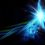# Work-Energy Theorem

The work-energy theorem is a very important and useful theorem in physics and here I will show you how we get that specific formula in full understanding.

Energy can help us solve many things however, it is a very abstract concept that we have no clue about. While the change in energy of two objects can be the same as the roll down a ramp and different inclinations, the rate at which the kinetic energy gets converted from potential energy is different and that is what we can start analyzing first.

$dK/dt = d(0.5mv^2)/dt = 2*0.5mv(dv/dt) = mv(dv/dt)$

We let $m$ be a constant as we assume that the object itself is not moving at any high speeds and has negligible relativistic effects. That final solution can also be written as:

$mv(dv/dt) = Fv$

We call this expression power and it is exactly what we have been talking about, the rate at which energy is expended to some object such as a car in order to make it move and do work.

$dK/dt = F(dx/dt)$

We can cancel the $dt's$ from both sides.

$dK = Fdx$

$∫ dK = ∫ Fdx$

$K(2) - K(1) = ∫ Fdx$

And that is the work-energy theorem. It is very important and very useful to use in physics, specifically in areas such as gravitational fields, electric fields, and oscillatory motion.Note by Raghu Alluri
2 years ago

This discussion board is a place to discuss our Daily Challenges and the math and science related to those challenges. Explanations are more than just a solution — they should explain the steps and thinking strategies that you used to obtain the solution. Comments should further the discussion of math and science.

When posting on Brilliant:

• Use the emojis to react to an explanation, whether you're congratulating a job well done , or just really confused .
• Ask specific questions about the challenge or the steps in somebody's explanation. Well-posed questions can add a lot to the discussion, but posting "I don't understand!" doesn't help anyone.
• Try to contribute something new to the discussion, whether it is an extension, generalization or other idea related to the challenge.

MarkdownAppears as
*italics* or _italics_ italics
**bold** or __bold__ bold
- bulleted- list
• bulleted
• list
1. numbered2. list
1. numbered
2. list
Note: you must add a full line of space before and after lists for them to show up correctly
paragraph 1paragraph 2

paragraph 1

paragraph 2

[example link](https://brilliant.org)example link
> This is a quote
This is a quote
    # I indented these lines
# 4 spaces, and now they show
# up as a code block.

print "hello world"
# I indented these lines
# 4 spaces, and now they show
# up as a code block.

print "hello world"
MathAppears as
Remember to wrap math in $$ ... $$ or $ ... $ to ensure proper formatting.
2 \times 3 $2 \times 3$
2^{34} $2^{34}$
a_{i-1} $a_{i-1}$
\frac{2}{3} $\frac{2}{3}$
\sqrt{2} $\sqrt{2}$
\sum_{i=1}^3 $\sum_{i=1}^3$
\sin \theta $\sin \theta$
\boxed{123} $\boxed{123}$